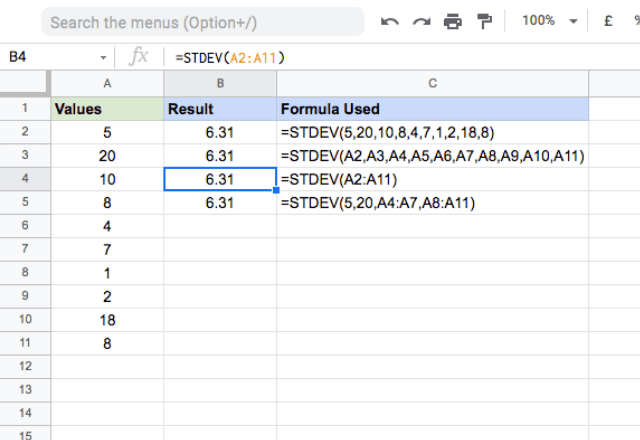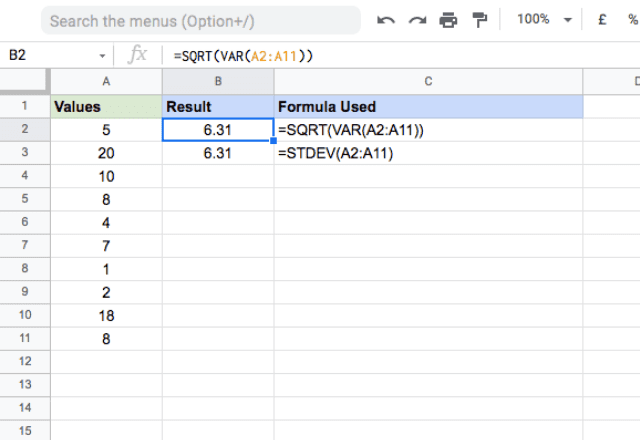# How to use the STDEV formula in Google SheetsGoogle Sheets is a great tool for data analysis tasks. Oftentimes, a key part of this involves statistical analysis, which can help you understand the nature of the dataset. Standard deviation is one of a variety of statistical measures that helps you quantify the degree of variation or dispersion of a set of data values. The STDEV function in Google Sheets is an effective and easy way to measure standard deviation.

=STDEV(value1, [value2, …])
• value1 – is the first value or reference to the range of the dataset.
• value2, … – [ OPTIONAL ] – are additional values or references to ranges that contain values you want to include in the dataset.

### What is standard deviation

I’ll take a moment to talk a bit more about standard deviation, in case you’re not familiar with it. As I mentioned previously, standard deviation measures the dispersion of a dataset. In other words, it indicates how far the data points are from the overall average of the dataset.

To illustrate this, consider a simple example where you have three numbers: 19, 20, and 21. The average of these three numbers is 20, and they’re all very close to the average. Now consider another dataset: 10, 20, and 30. Again, the average of these is 20, but there’s much more variation. In these two examples, the first dataset has a lower standard deviation, and the standard deviation of the second group is higher.

### Usage: STDEV formula in Google Sheets

Now that you understand the concept of standard deviation, I’ll show how to use the STDEV formula on a sample dataset to further reinforce your understanding of how to calculate it in Google Sheets.

Please take a look at the following snapshot.The first thing you’ll notice is that in all four instances above, you get the same result. This makes sense, since I’m using the same data each time. The point here is to demonstrate the different ways you can input data into the STDEV function.

The first example takes direct numeric values and returns the calculated standard deviation. The second demonstrates that the STDEV formula is also capable of using references to cells that store numbers. In the third example, instead of sending multiple values or references, I’ve entered a single range that contains all the values from A2 through A11. This is the easiest method, since you only have to input a single range. In the final example, I typed a combination of direct numeric values and range references.

As you can see, regardless of the approach you take with the formula, the output will be the same.

### Notes

• STDEV will return an error if any of the parameter values are strings.
• This formula calculates the standard deviation for a sample. If you need to do it for a population instead, you can use the STDEVP formula.
• The formula may return a #DIV/0! error if there are not at least two value arguments.
• If you’re familiar with statistics, you’ll know that standard deviation is just the square root of the variance, another important metric in statistics. You can calculate this using the VAR formula in Google Sheets. The image below illustrates this concept.### STDEV formula in Google Sheets

That’s it. That’s how you use the STDEV formula in Google Sheets.